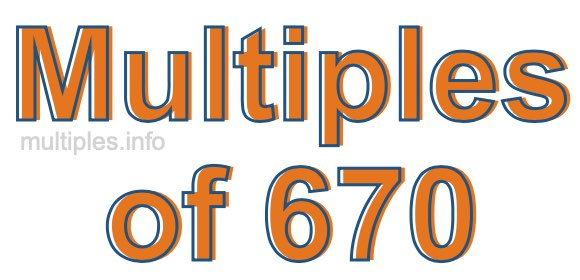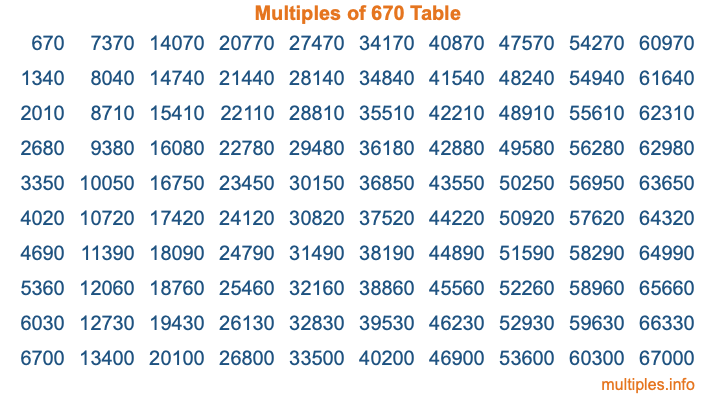Multiples of 670Welcome to the Multiples of 670 page. Here we will first teach you everything you will ever need to know about the multiples of 670, and then give you a study guide summary of everything we taught you to make sure you remember it all. Use this page to look up facts and learn information about the multiples of 670. This page will make you a multiples of six hundred seventy expert!

Definition of Multiples of 670
Multiples of 670 are all the numbers that when divided by 670 equal an integer. Each of the multiples of 670 are called a multiple. A multiple of 670 is created by multiplying 670 by an integer.

Therefore, to create a list of multiples of 670, you start with 1 multiplied by 670, then 2 multiplied by 670, then 3 multiplied by 670, and so on for as long as you want. Thus, the list of the first five multiples of 670 is 670, 1340, 2010, 2680, and 3350. To see a larger list of multiples of 670, see the printable image of Multiples of 670 further down on this page. We also have a category where you can choose any nth multiple of 670.

Multiples of 670 Checker
The Multiples of 670 Checker below checks to see if any number of your choice is a multiple of 670. In other words, it checks to see if there is any number (integer) that when multiplied by 670 will equal your number. To do that, we divide your number by 670. If the the quotient is an integer, then your number is a multiple of 670.

Is  a multiple of 670?

Least Common Multiple of 670 and ...
A Least Common Multiple (LCM) is the lowest multiple that two or more numbers have in common. This is also called the smallest common multiple or lowest common multiple and is useful to know when you are adding our subtracting fractions. Enter one or more numbers below (670 is already entered) to find the LCM.

Check out our LCM Calculator if you need more details about the Least Common Multiple or if you need the LCM for different numbers for adding and subtraction fractions.

nth Multiple of 670
As we stated above, 670 is the first multiple of 670, 1340 is the second multiple of 670, 2010 is the third multiple of 670, and so on. Enter a number below to find the nth multiple of 670.

th multiple of 670

Multiples of 670 vs Factors of 670
670 is a multiple of 670 and a factor of 670, but that is where the similarities end. All postive multiples of 670 are 670 or greater than 670. All positive factors of 670 are 670 or less than 670.

Below is the beginning list of multiples of 670 and the factors of 670 so you can compare:

Multiples of 670: 670, 1340, 2010, 2680, 3350, etc.

Factors of 670: 1, 2, 5, 10, 67, 134, 335, 670

As you can see, the multiples of 670 are all the numbers that you can divide by 670 to get a whole number. The factors of 670, on the other hand, are all the whole numbers that you can multiply by another whole number to get 670.

It's also interesting to note that if a number (x) is a factor of 670, then 670 will also be a multiple of that number (x).

Multiples of 670 vs Divisors of 670
The divisors of 670 are all the integers that 670 can be divided by evenly. Below is a list of the divisors of 670.

Divisors of 670: 1, 2, 5, 10, 67, 134, 335, 670

The interesting thing to note here is that if you take any multiple of 670 and divide it by a divisor of 670, you will see that the quotient is an integer.

Multiples of 670 Table
Below is an image of the first 100 multiples of 670 in a table. The table is in chronological order, column by column. The first column has the first ten multiples of 670, the second column has the next ten multiples of 670, and so on.The Multiples of 670 Table is also referred to as the 670 Times Table or Times Table of 670. You are welcome to print out our table for your studies.

Negative Multiples of 670
Although not often discussed or needed in math, it is worth mentioning that you can make a list of negative multiples of 670 by multiplying 670 by -1, then by -2, then by -3, and so on, to get the following list of negative multiples of 670:

-670, -1340, -2010, -2680, -3350, etc.

Multiples of 670 Summary
Below is a summary of important Multiples of 670 facts that we have discussed on this page. To retain the knowledge on this page, we recommend that you read through the summary and explain to yourself or a study partner why they hold true.

There are an infinite number of multiples of 670.

A multiple of 670 divided by 670 will equal a whole number.

670 divided by a factor of 670 equals a divisor of 670.

The nth multiple of 670 is n times 670.

The largest factor of 670 is equal to the first positive multiple of 670.

670 is a multiple of every factor of 670.

670 is a multiple of 670.

A multiple of 670 divided by a divisor of 670 equals an integer.

670 divided by a divisor of 670 equals a factor of 670.

Any integer times 670 will equal a multiple of 670.

Multiples of a Number
Here you can get the multiples of another number, all with the same attention to detail as we did for multiples of 670 on this page.

Multiples of
Multiples of 671
Did you find our page about multiples of six hundred seventy educational? Do you want more knowledge? Check out the multiples of the next number on our list!